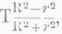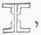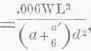Strength Of Materials, the resistance offered by the materials of construction to change of form or to fracture. 1. The resistance of materials to external forces tending to overcome their cohesion is classified, according to their forms, as follows :

 Longitudinal. Compressing, resisting pulling asunder. Tensile, resisting crushing. Bending, resisting cross breaking. Transverse.... Shearing, resisting cutting across. Torsional, resisting twisting or wrenching.

Two or more of these forms of resistance are sometimes called into action simultaneously, as in the case of the crank of a steam engine, which tends to break the shaft both by a transverse strain and by torsion. 2. The "ultimate strength" is the maximum resistance offered to rupture. The " proof strength " is a less degree of resistance, which the body may safely offer when tested. The "working load " is some fractional part of the ultimate strength which may be selected as giving perfect safety against anticipated strains for an indefinite period. 3. The "factor of safety" is the ratio of the ultimate strength to the working load. The following are minimum values of this quantity adopted in what is generally considered good practice, under "dead " and " live " loads, and where the latter are'liable to be accompanied by heavy shocks :

 MATERIAL. Dead load. Live load. Shock. Wrought iron ............... 3 5 8 Steel........................ 3 6 8 Cast iron ............... 4 8 10 Timber ................ 4 8 10 Masonry ..................... 6 10 ••

4. The proof strength is usually, and should be always, below the elastic limit, i. e., the point at which set becomes proportional, or nearly so, to the distortion produced by the applied force. It is generally about one half or one third the ultimate strength. 5. Tensile resistance, or tenacity, is determined by experiment for each material. The ultimate strength or breaking load of any piece is measured by the product of the area of fractured section into the tenacity of the material of which it is composed; i. e., P=TK, and K=where P represents the breaking force, T the tenacity of the material, and K the area of section. Values of T are given in the accompanying table of coefficients of resistance. The very best grades should have values 20 per cent, higher. P and T are taken in pounds upon the square inch. 6. When thin cylinders are exposed to internal pressure, as in steam boilers, steam cylinders, etc, the bursting pressure may be determined by multiplying the thickness of the shell by the tenacity of the material, as given above, and dividing by the semi-diameter. To ascertain the thickness, the pressure and the diameter of the cylinder being given, multiply the pressure by the semi-diameter, and divide by the tenacity of the material as given in the table; or P=and t=where P = pressure, t = thickness, T = tenacity, and r = radius of the cylinder. If d = - diameter, P=and t =.

Where the joints are double-riveted, the strength at the joints is usually about 0.7 that of the solid plate; single-riveted joints have 0.56 the strength of a solid plate. The mean strength of single-riveted boilers, where the joints are properly shifted, or "broken," is nearly or quite five eighths that of solid plate. A sphere will bear twice as much internal pressure as a cylinder of the same diameter. A thick cylinder is not as strong relatively as a thin cylinder of the same material, and no cylinder, however thick, can withstand an internal pressure exceeding its limit of tenacity, T, as given above. The rule for calculating the strength of a thick cylinder is expressed algebraically thus:

P=andwhere r = internal radius, R==the external radius, and other values as before. For thick spheres, P=and.

7. The stayed surfaces are usually the strongest parts of a steam boiler. The following formula gives the proper distance between stays, where t = thickness of plate, P = the pros-sure in pounds per square inch, and F= the factor of safety: d=P=F.

8. Bolts, rivets, and lugs are usually exposed to shearing strain. The resistances to shearing of the most commonly used materials are to be taken as equal to the tensile strength.

Where shearing is to be resisted, the parts should be fitted with" great care, to avoid the possibility of cutting, and to insure that all parts of the cross section attacked shall resist the shearing force as nearly as possible together. Where a pin is fitted but not forced into its socket, the resistance to shearing is taken as three fourths of that due the section exposed to rupture. 9. Crushing is resisted by any given material with a force that varies very greatly with the form given it. Very short columns or compact masses resist very high crushing strains, in consequence of the resistance offered by their particles to dispersion, as well as by their cohesion. Tall columns first bend and then break under a comparatively slight force. The figures in column C of the table give the resistance to crushing when bending does not occur. Seasoned timber has nearly twice as great resistance to crushing as green. Steel should not be used under pressure exceeding its compressive elasticity, which, in tool steel, is about 50,000 lbs. to the square inch.

Wrought iron should not be used under pressure exceeding 25,000 lbs. to the square inch. 10. For tall columns, the following formulas were proposed by Prof. Eaton Hodgkinson:

 MATERIALS. Rounded ends. Flat ends. Solid cylindrical cast-iron col- W = (D3.7C/L1.7)149 w = 44.2(D3.55/L1.7) Hollow cylindrical cast-iron columns...... W = 13(D3.76-d3.76/L1.7) w = 44.3(D3.55-d3.55/L1.7) Solid cylindrical wrought-iron columns...... W = 42(D3.76/L2) W = 133.7(D3.55/L2) Solid square pillar of Dantzic oak.......... .................................... W = I0.9(D4/L2) Solid square pillar of red pine......... .................. W = 7.8(D4/L2)

In these formulas W = crushing weight in tons, 1) = outside diameter in inches, d = inside diameter in inches, L = length in feet, and 1) <'4L; i. c, the columns are more than 30 diameters in length. Prof. Rankine gives P =for tall columns. P = crushing load in pounds; S = sectional area in square inches; I = length and D = external diameter, both being in the same units of measure, whether feet or inches. The following are the values of f and a:

 MATERIALS. Value of f. Value of a. Forms of column. Cast iron.......... 80.000 1/400 Hollow cylinder. Wrought iron..... 36,000 1/3000 Solid rectangle. " " ............... 36.000 1/6000 Thin square tube " " ............ 36.000 1/2250 Solid cylinder. " " .. ...... 36,000 1/4500 Thin cylinder. " " ........ 36.000 1/1500 Angle iron. " " . . . . . 36,000 l/1500 + shaped. Best American wrought iron.. . 50.000 ....... For all shapes. Timber........... 6,500 1/250 Solid rectangle.

This formula is frequently designated as Gordon's, having been deduced by Gordon from Hodgkinson's experiments. Multiply the value of a, as given in the table, by 4 for columns rounded or jointed at both ends, and by 2 where fixed at one end, rounded at the other. Connecting rods of steam engines are calculated as pillars rounded at both ends. Piston and pump rods are considered as fixed at one end, free at the other. 11. The collapsing of boiler flues was made the subject of a series of experiments by Mr. Fairbairn, and the following formula was deduced: P=806,000where P = collapsing pressure in pounds per square inch, t = thickness of iron in flues, L = length of flue in feet, and d = its diameter in inches. When the flue is strengthened by angle-iron rings, as is sometimes done with long flues, L is taken as the distance between the rings. This formula has not been verified for short flues of great diameter, or for exceptional proportions. A slight deviation from a truly cylindrical form considerably reduces the strength of the flues; t2- is generally taken instead of t'2'10. Elliptical flues, having a major diameter a and a minor diameter d, are of equal strength with a cylindrical flue of the diameter. 12. The transverse strength of beams may be calculated by the following formulas :for beams fixed at one end and loaded at the other.where fixed at one end and uniformly loaded.where supported at both ends and loaded at centre.where fixed at both ends and loaded at centre.where supported at both ends and uniformly loaded.where fixed at both ends and uniformly loaded.

Here W = breaking weight in pounds, K = a coefficient which varies with every change in form of cross section of the beams, d = depth of beam in inches, b = breadth in inches, A = area of cross section of the beam at point of rupture in square inches, and L = length between supports in feet. The values of K given in the table, where the beams are of rectangular section, fixed at one end and loaded at the other, are obtained from various sources. 13. For other than rectangular sections the following may be taken as the values of K for cast iron: Shape,; value, K = 500. Shape,equal flanges; value, K = 520. Fairbairn,; value, K = 580. Hodgkinson,value, K = 850. The following values are given for wrought iron: rolled rails,600; Fairbairn's riveted beam,900; box beam,1,000. 14. For the wrought-ironbeam, when supported at both ends and uniformly loaded, the formula W =is used by some American manufacturers. D= depth in feet; a= area of flange in inches, a= that of "stem" or web; S = stress per square inch of area,in tons. The deflection, Swhere the load is applied at the middle, and S'=when applied uniformly. The depth D is measured between the centres of gravity of the flanges. In such beams it is customary to allow as maxima 10,000 lbs. per square inch in tension and 6,000 to 8,000 in compression. Deflection should not exceed 1/30 of an inch per foot of length, in any structure. 15. Torsional strength is computed by the formula W =where "W = breaking weight in pounds, D = diameter of shaft in inches, and R = length of lever arm in feet. The coefficient S' is very nearly proportional to the tenacity of the material, where the torsion is equal in degree. 16. Resilience is a term introduced by Dr. Young. It is measured by the amount of work performed in producing the maximum strain which a given body is capable of sustaining, and is the quality of primary importance where shocks are to be sustained. Mallet's coefficient of resilience is the half product of the maximum resistance into the maximum extension. But for tough metals it is equal approximately to two thirds the product of the ultimate strength of the material by the distance through which the body yields before the straining force. For very brittle materials it is measured by half that product. No material can resist the shock of a body in motion, unless it is capable of offering resilience equal to the amount of work performed in setting that body in motion at the given velocity; i. e., equal to the amount of energy stored in the moving mass at the instant of striking.

In predicting the effect of shock, therefore, it becomes necessary to know the amount of energy stored in the moving body and the resilience of the resisting material. To meet a violent shock successfully, resilience, rather than mere strength, must be secured. As an instance, it is found that wrought iron of comparatively low tenacity but great toughness, capable of stretching considerably before fracture, is far superior to steel for armor for iron-clad ships; the latter has much greater strength, but also greater brittleness. Such calculations are not usually made in designing. Immunity from the injurious effect of shock is secured by the use of a large factor of safety in proportioning parts exposed to them, by care during construction in the selection of tough resilient materials, and in management by carefully adjusting all parts, and applying the load so as to avoid jarring action as far as possible. 17. If a weight, acting as a steady load, produces a given deflection or change of dimensions, it will require but half that weight suddenly applied to produce a similar effect, whether it be fracture or a stated alteration of form. The extension of ordinary wrought iron within its limit of elasticity is about .0001 per ton per square inch of section.

The amount of extension before fracture by tension is given, with the tinest quality of wrought iron, at 20 per cent., with medium quality 16 per cent., and it runs in some irons as low as 4 per cent. Cast iron of fair quality is elongated but a fraction of 1 per cent. 18. The extension of steel varies with the amount of carbon, and nearly inversely as its tenacity. The following table is taken in part from Traut wine's "Engineer's Pocket Book:1'